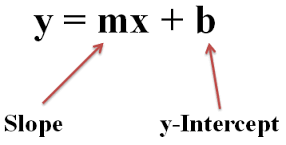# Slope-Intercept FormThis is the formula for finding the slope-intercept. Slope-intercept represents the equation of a line. Find the slope , x and y-intercept of the problem below and plug them into the formula and solve for the slope-intercept problem.

## Explaination

Answer: y=2x+3 The way to find the answer for the problem above is you start of finding slope which you can do by picking a point like for example you could start on the point (-1,1) then try to get to the point (3,0) and the rise 2.and the run is 1 so the slope is 2. Then to find B which is the y-intercept you use the point (3,0) for your x and y-intercept.